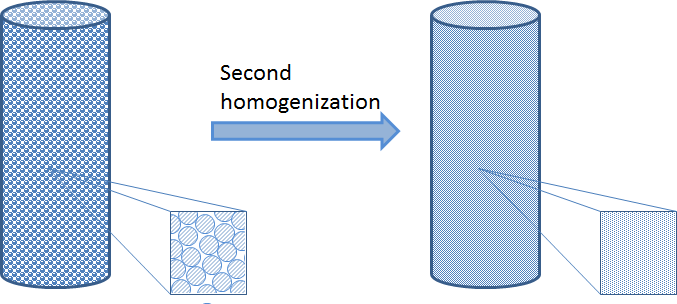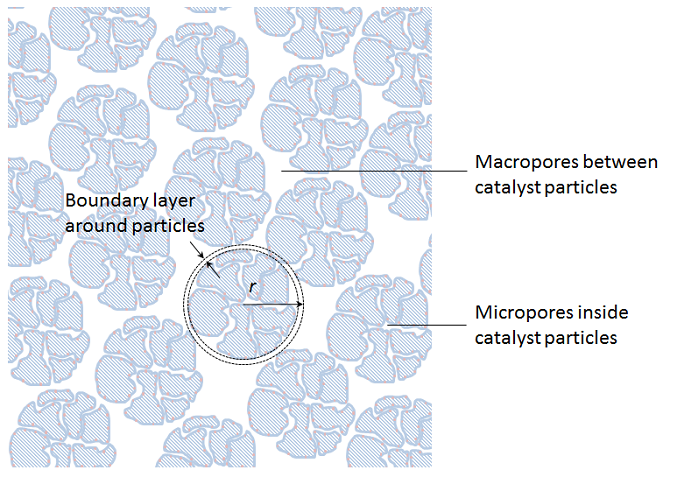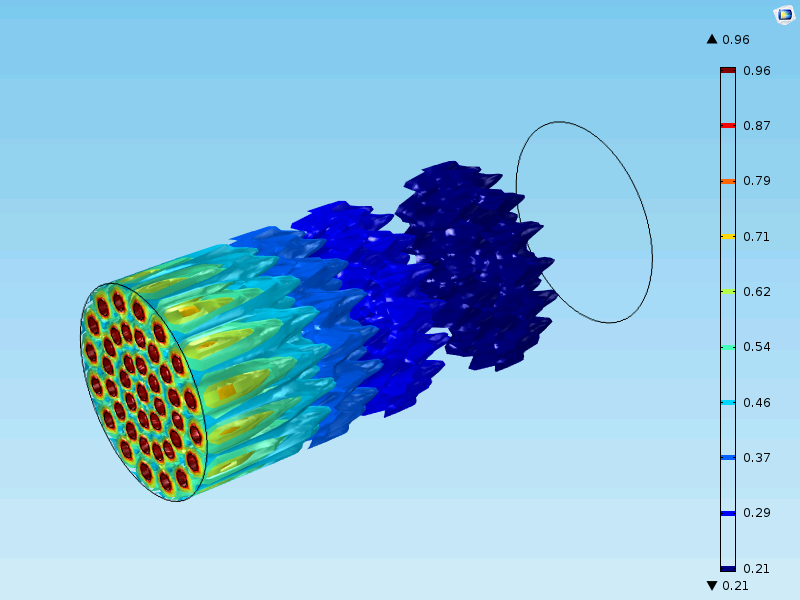# 多相催化建模方法

2015年 2月 3日

### 主要步骤

1. 将反应物从混合物的主体输送到催化剂颗粒
2. 将催化剂颗粒孔隙中的反应物输送到活性位点
3. 反应物对活性位点的吸附
4. 反应物反应，形成吸附产物
5. 产品从活性位点的解吸
6. 催化颗粒孔隙中的产物从颗粒中移出
7. 产品从颗粒输送到大部分混合物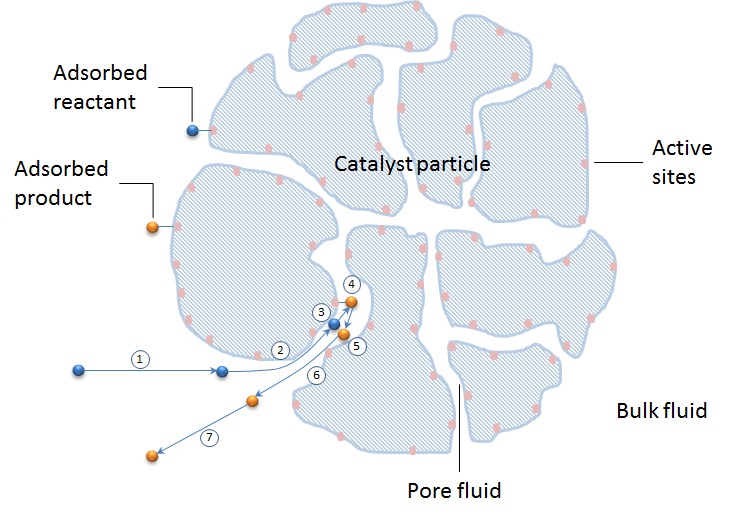### 吸附-解吸模型

1. 对于不同的吸附位点和不同的表面覆盖率，吸附在能量上是均匀的
2. 假设吸附的物质形成单层
3. 吸附的分子之间没有相互作用

Freundlich 等温线是基于这样的假设：吸附是不均匀的，一些位点具有较高的吸附系数，因此首先被分子覆盖。这些位点有更多的吸附放热。吸附热与表面覆盖率呈对数关系。Temkin 等温线可以通过假设吸附热与表面覆盖率的线性关系来获得。其他吸附模型（如 BET 等温线）也包括多层分子，这些分子必须在其描述中包括物理吸附。

### 表面反应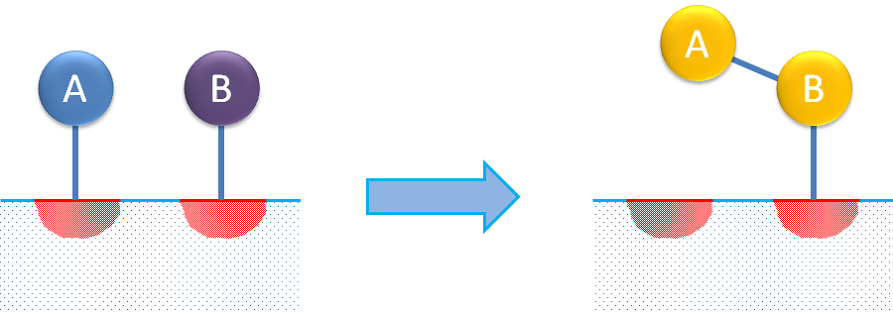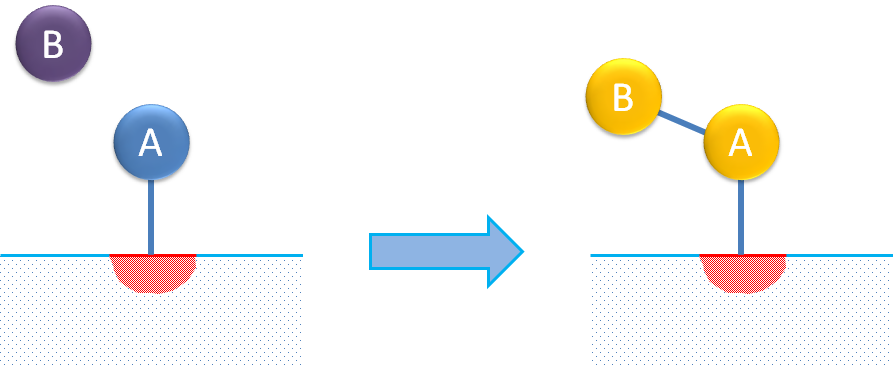(1)

A = B

(2)

\begin
{array}{l} A + S = AS(ads)\\
BS(ads) = B + S \end{array}\\

(3)

{r_A}= \frac{{\theta \left( {{c_A} – \frac{{
{c_B}}}{K}} \right)}}{{\left( {{k_1} + {k_2}{c_A} + {k_3}{c_B}} \right)}}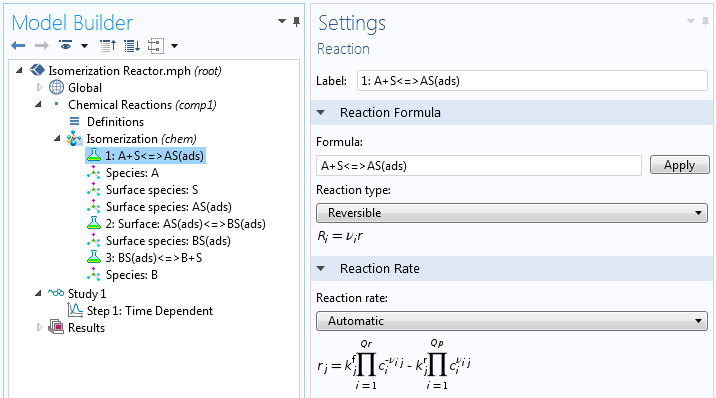### 输运步骤

1. 催化剂微观结构的详细描述
2. 对每个颗粒的均质化描述
3. 详细描述大多数颗粒的结构，其中单个颗粒的描述是均质的
4. 颗粒床的均质化与颗粒的均质化描述相结合

#### 微观尺度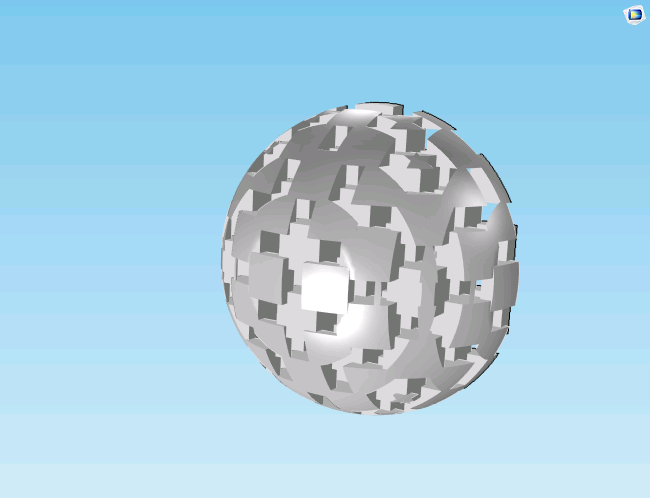(4)

\frac{{\partial
{c_i}}}\partial t + \nabla \cdot \bf{N_i} = 0

(5)

${{\bf{N}}_i} \cdot {\bf{n}} = – {R_i}$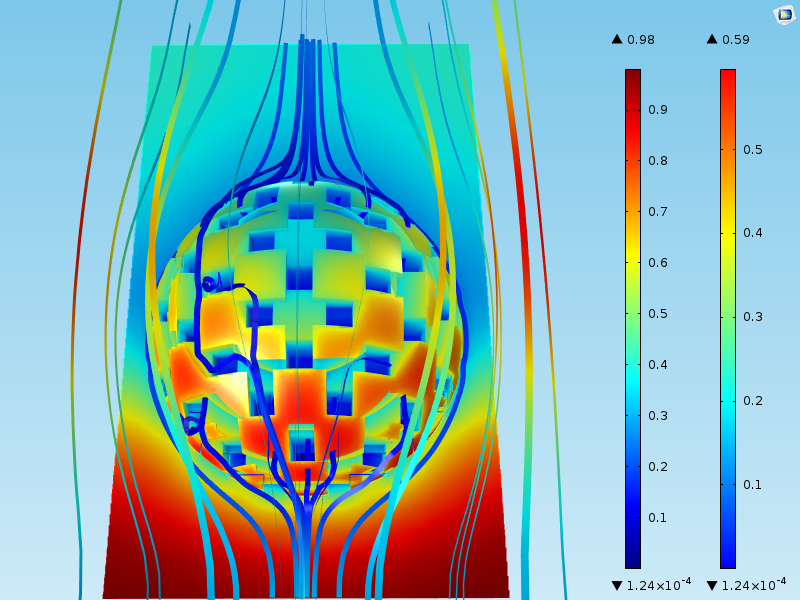#### 微观尺度上的均质化

(6)

\frac{{\partial {c_i}
}}\partial t + \nabla \cdot \bf{N_i} –
{S_a}{R_i} = 0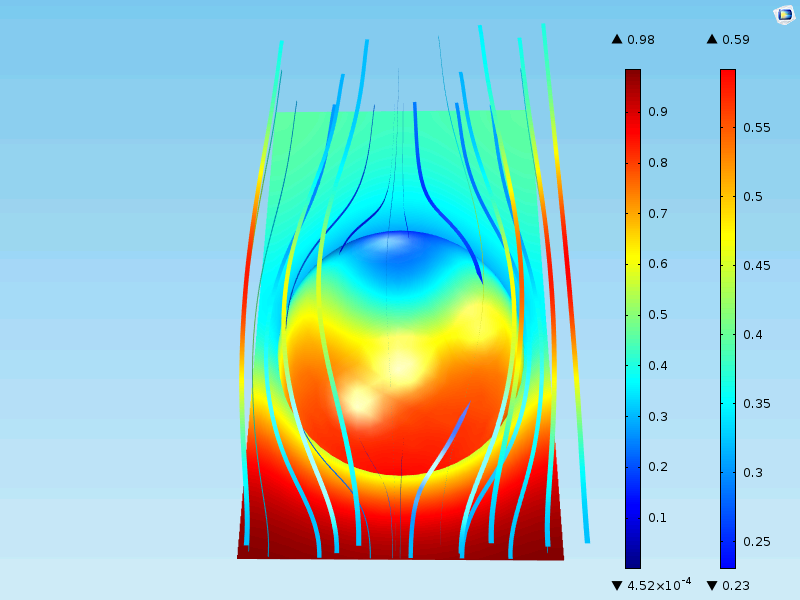#### 在宏观尺度上详细描述大多数均质颗粒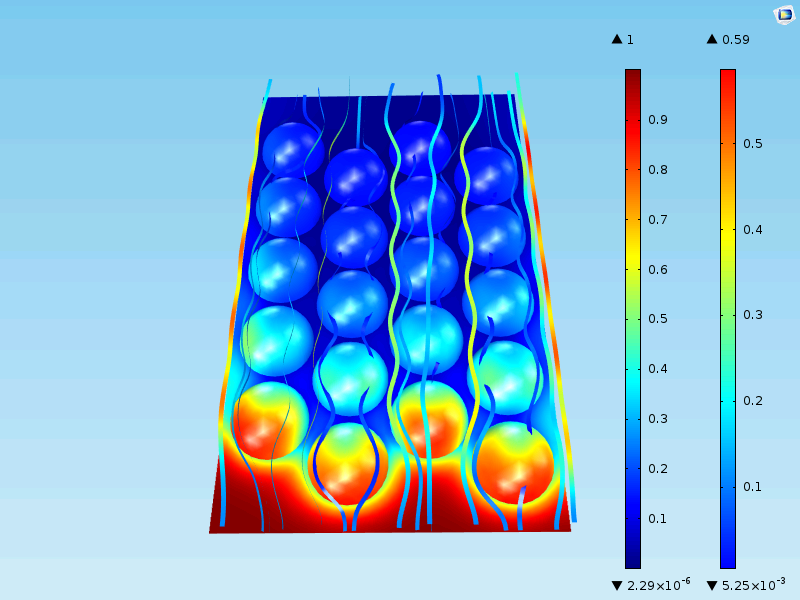#### 宏观尺度上的均质化描述